# Chapter 3 Newtons Law NEXT Newtons Laws Newtons

• Slides: 20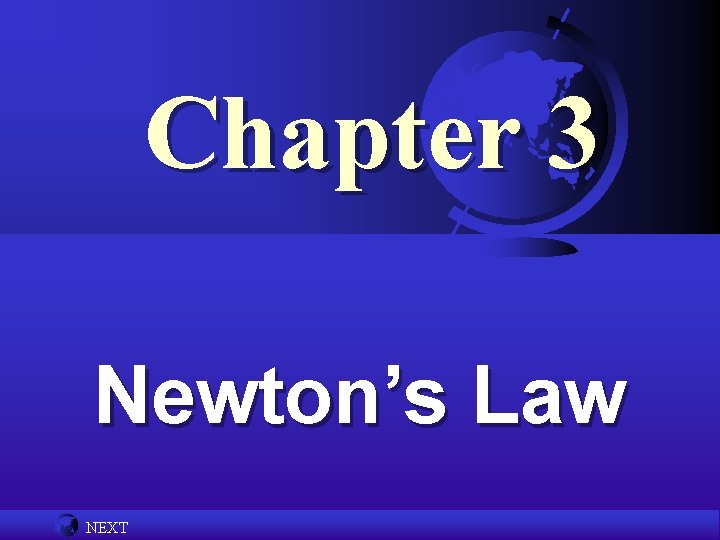Chapter 3 Newton’s Law NEXT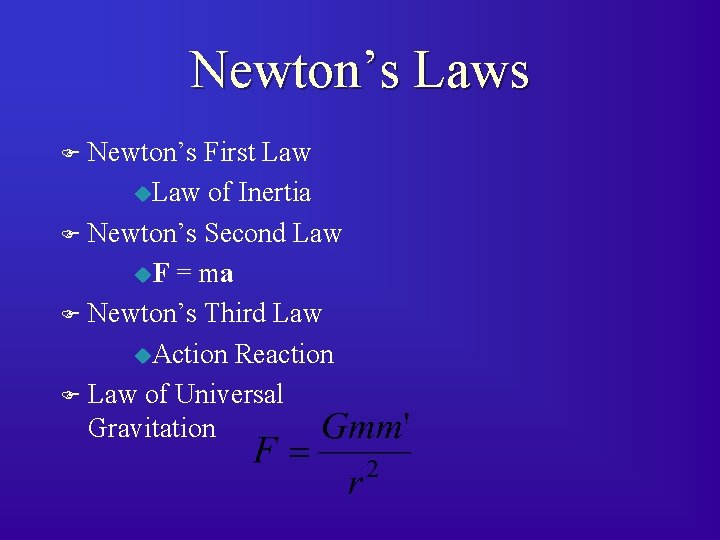Newton’s Laws Newton’s First Law u. Law of Inertia F Newton’s Second Law u. F = ma F Newton’s Third Law u. Action Reaction F Law of Universal Gravitation F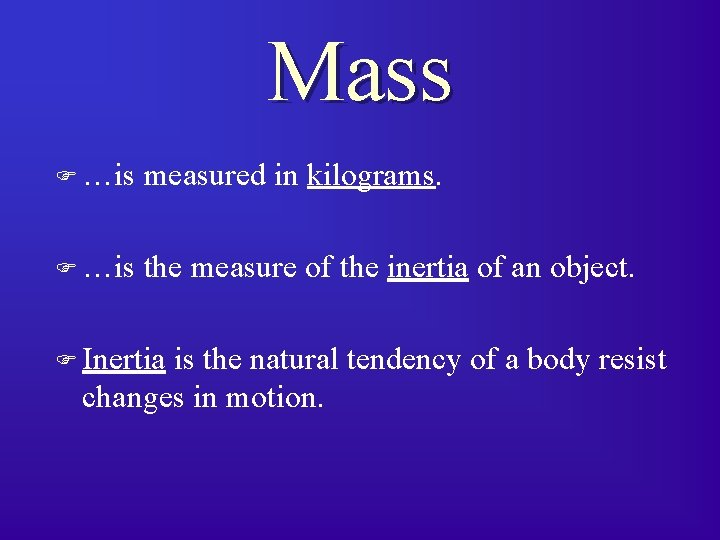Mass F …is measured in kilograms. F …is the measure of the inertia of an object. F Inertia is the natural tendency of a body resist changes in motion.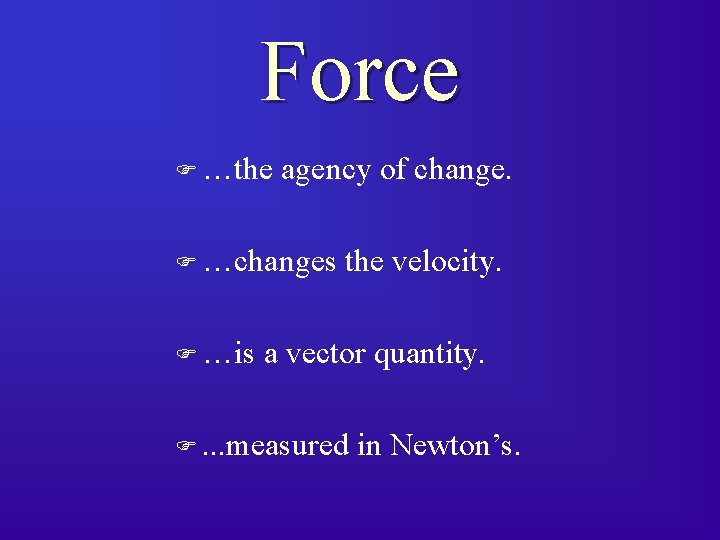Force F …the agency of change. F …changes F …is the velocity. a vector quantity. F. . . measured in Newton’s.Ancient View of the Cosmos F F F Universe is 2 -D – All celestial objects attached to a sphere. Celestial Sphere is close – Climb a high mountain and touch the sky Celestial objects are self-luminous Earth is the center of the universe Objects move on perfect circlesAristotelian Universe Terrestrial Realm o Composition predicts motion natural tendencies Fire and Air tend to rise Earth and Water tend to sink o Overall tendency to seek rest o Objects following tendencies require no force o Objects are corruptible (changing)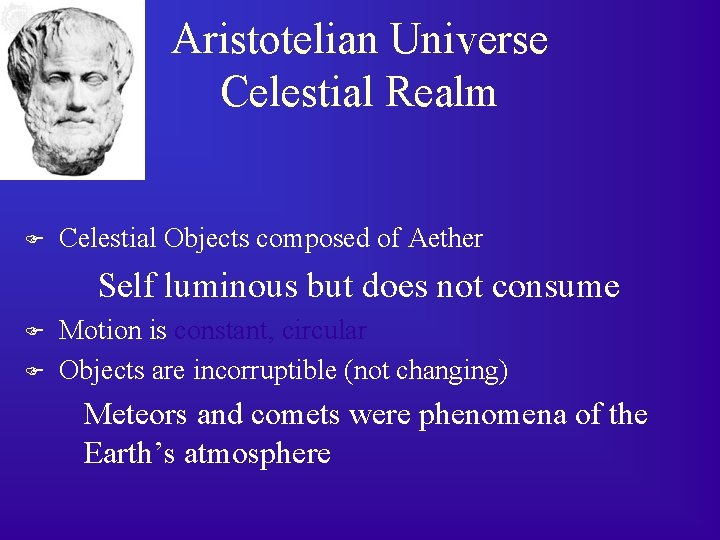Aristotelian Universe Celestial Realm F Celestial Objects composed of Aether Self luminous but does not consume F F Motion is constant, circular Objects are incorruptible (not changing) Meteors and comets were phenomena of the Earth’s atmosphere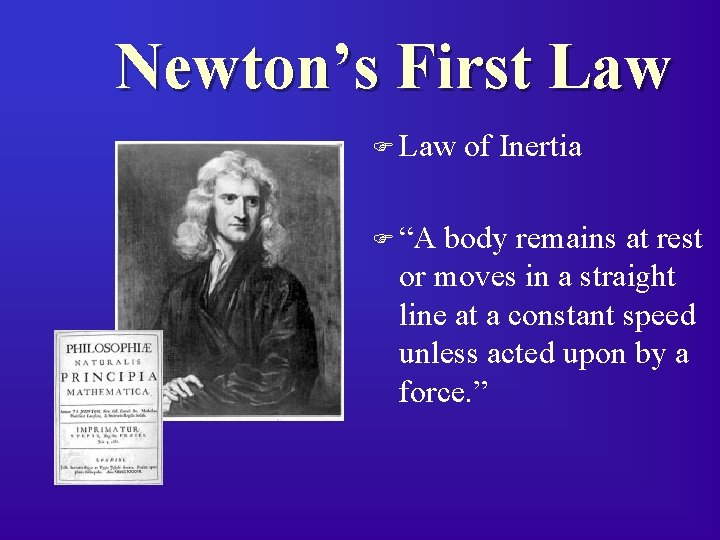Newton’s First Law F “A of Inertia body remains at rest or moves in a straight line at a constant speed unless acted upon by a force. ”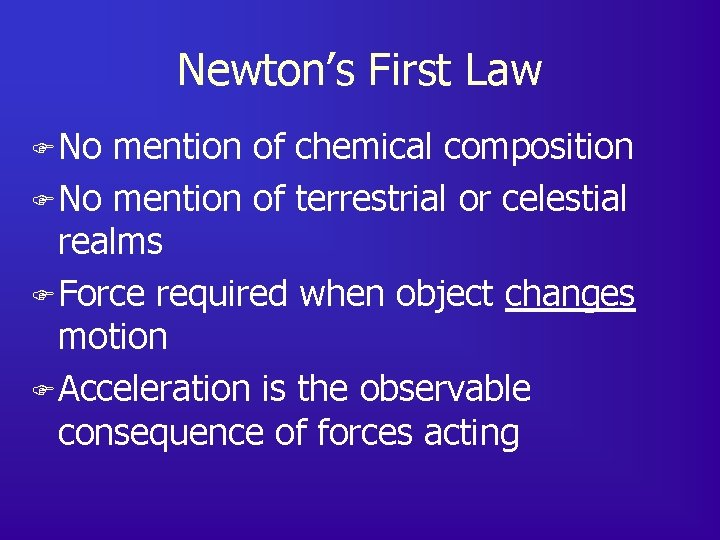Newton’s First Law F No mention of chemical composition F No mention of terrestrial or celestial realms F Force required when object changes motion F Acceleration is the observable consequence of forces actingNewton’s Second Law The Sum of the Forces acting on a body is proportional to the acceleration that the body experiences SF a S F = (mass) a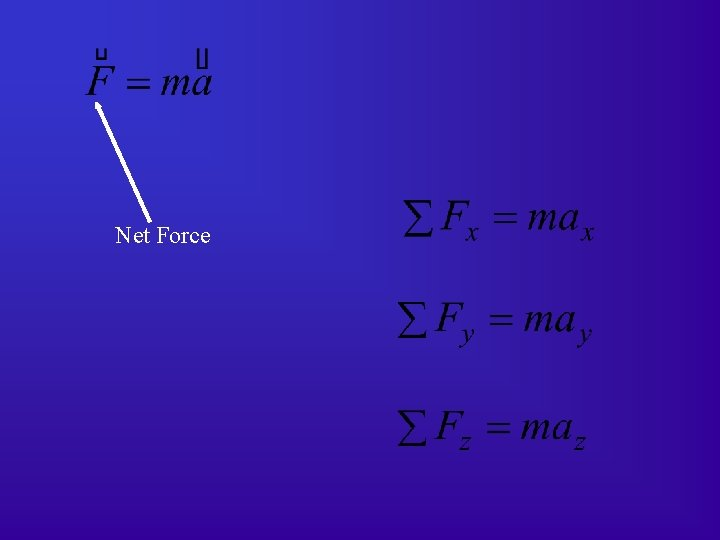Net Force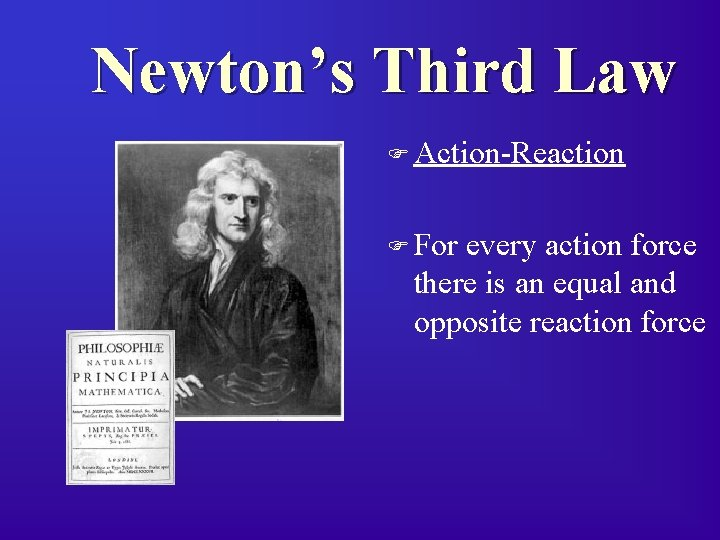Newton’s Third Law F Action-Reaction F For every action force there is an equal and opposite reaction forceThe Law of Gravity F Every mass exerts a force of attraction on every other mass. F The math… FG = 6. 67 10 -11 N·m 2/kg 2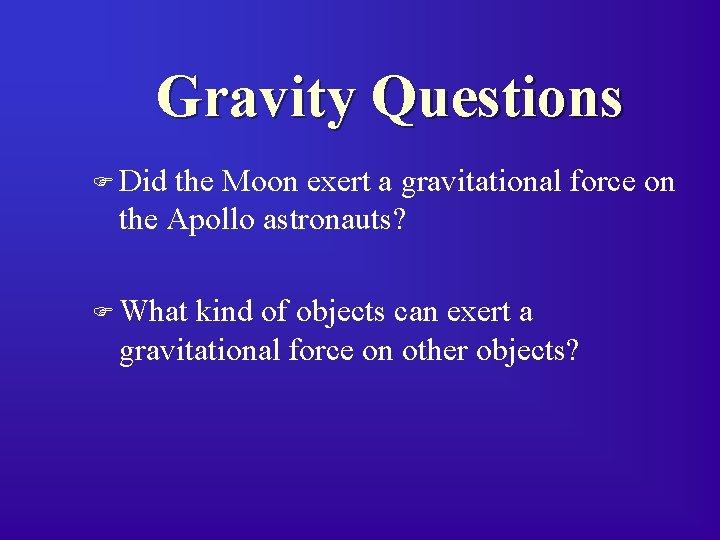Gravity Questions F Did the Moon exert a gravitational force on the Apollo astronauts? F What kind of objects can exert a gravitational force on other objects?Gravity Questions F The constant G is a rather small number. What kind of objects can exert strong gravitational forces? F If the distance between two objects in space is doubled, then what happens to the gravitational force between them?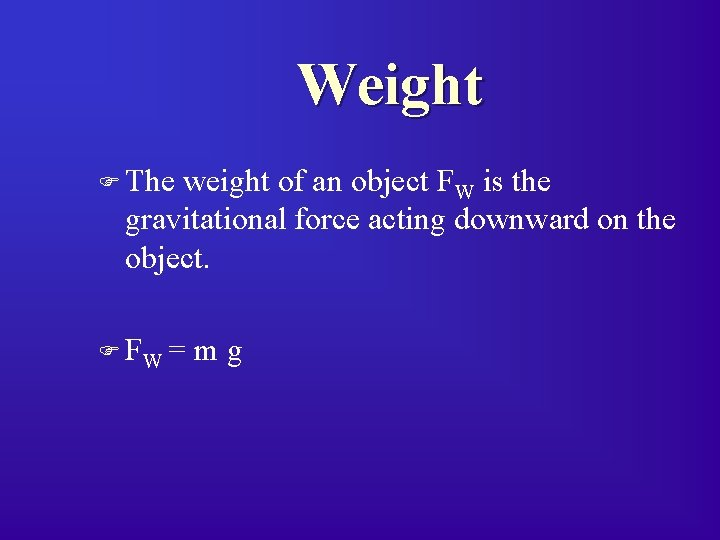Weight F The weight of an object FW is the gravitational force acting downward on the object. F FW = mgTension (Tensile Force) F Tension is the force in a string, chain or tendon that is applied tending to stretch it. F FTNormal Force F The normal force on an object that is being supported by a surface is the component of the supporting force that is perpendicular to the surface. F FN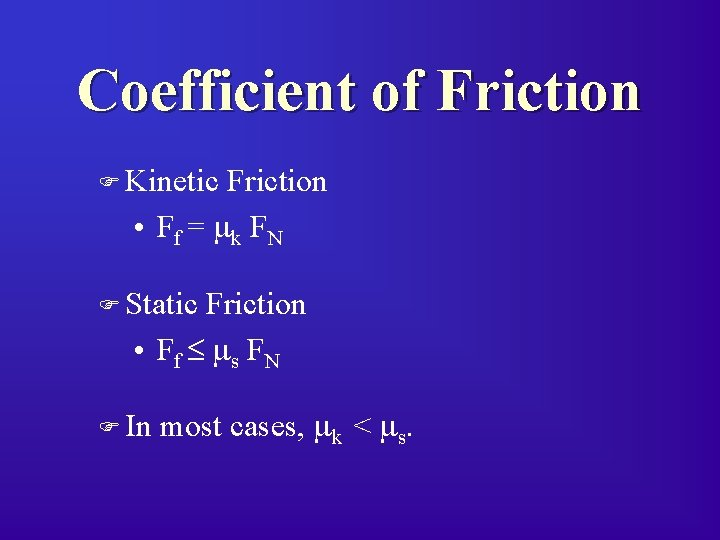Coefficient of Friction F Kinetic Friction • Ff = mk FN F Static Friction • Ff ms FN F In most cases, mk < ms.On to problems. . .